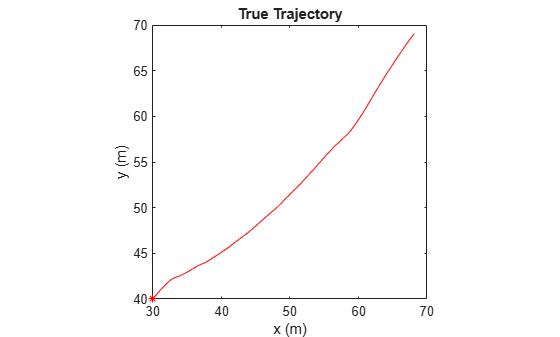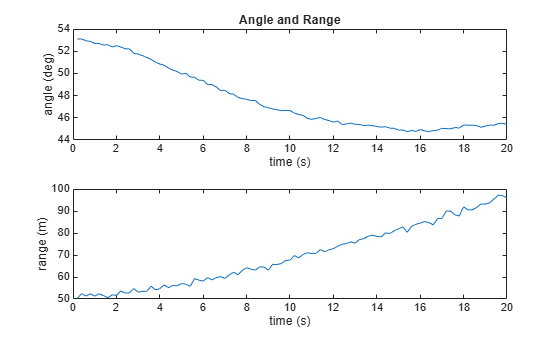## Extended Kalman Filters

When you use a filter to track objects, you use a sequence of detections or measurements to estimate the state of an object based on the motion model of the object. In a motion model, state is a collection of quantities that represent the status of an object, such as its position, velocity, and acceleration. Use an extended Kalman filter (`trackingEKF`) when object motion follows a nonlinear state equation or when the measurements are nonlinear functions of the state. For example, consider using an extended Kalman filter when the measurements of the object are expressed in spherical coordinates, such as azimuth, elevation, and range, but the states of the target are expressed in Cartesian coordinates.

The formulation of an extended Kalman is based on the linearization of the state equation and measurement equation. Linearization enables you to propagate the state and state covariance in an approximately linear format, and requires Jacobians of the state equation and measurement equation.

Note

If your estimate system is linear, you can use the linear Kalman filter (`trackingKF`) or the extended Kalman filter (`trackingEKF`) to estimate the target state. If your system is nonlinear, you should use a nonlinear filter, such as the extended Kalman filter or the unscented Kalman filter (`trackingUKF`).

### State Update Model

Assume a closed-form expression for the predicted state as a function of the previous state xk, controls uk, noise wk, and time t.

`${x}_{k+1}=f\left({x}_{k},{u}_{k},{w}_{k},t\right)$`

The Jacobian of the predicted state with respect to the previous state is obtained by partial derivatives as:

`${F}^{\left(x\right)}=\frac{\partial f}{\partial x}.$`

The Jacobian of the predicted state with respect to the noise is:

`${F}^{\left(w\right)}=\frac{\partial f}{\partial w}.$`

These functions take simpler forms when the noise is additive in the state update equation:

`${x}_{k+1}=f\left({x}_{k},{u}_{k},t\right)+{w}_{k}$`

In this case, F(w) is an identity matrix.

You can specify the state Jacobian matrix using the `StateTransitionJacobianFcn` property of the `trackingEKF` object. If you do not specify this property, the object computes Jacobians using numeric differencing, which is slightly less accurate and can increase the computation time.

### Measurement Model

In an extended Kalman filter, the measurement can also be a nonlinear function of the state and the measurement noise.

`${z}_{k}=h\left({x}_{k},{v}_{k},t\right)$`

The Jacobian of the measurement with respect to the state is:

`${H}^{\left(x\right)}=\frac{\partial h}{\partial x}.$`

The Jacobian of the measurement with respect to the measurement noise is:

`${H}^{\left(v\right)}=\frac{\partial h}{\partial v}.$`

These functions take simpler forms when the noise is additive in the measurement equation:

`${z}_{k}=h\left({x}_{k},t\right)+{v}_{k}$`

In this case, H(v) is an identity matrix.

In `trackingEKF`, you can specify the measurement Jacobian matrix using the `MeasurementJacobianFcn` property. If you do not specify this property, the object computes the Jacobians using numeric differencing, which is slightly less accurate and can increase the computation time.

### Extended Kalman Filter Loop

The extended Kalman filter loop is almost identical to the loop of Linear Kalman Filters except that:

• The filter uses the exact nonlinear state update and measurement functions whenever possible.

• The state Jacobian replaces the state transition matrix.

• The measurement jacobian replaces the measurement matrix.### Predefined Extended Kalman Filter Functions

The toolbox provides predefined state update and measurement functions to use in `trackingEKF`.

Motion ModelFunction NameFunction PurposeState Representation
Constant velocity`constvel`Constant-velocity state update model

• 1-D — `[x;vx]`

• 2-D — `[x;vx;y;vy]`

• 3-D — `[x;vx;y;vy;z;vz]`

where

• `x`, `y`, and `z` represents the position in the x-, y-, and z-directions, respectively.

• `vx`, `vy`, and `vz` represents the velocity in the x-, y-, and z-directions, respectively.

`constveljac`Constant-velocity state update Jacobian
`cvmeas`Constant-velocity measurement model
`cvmeasjac`Constant-velocity measurement Jacobian
Constant acceleration`constacc`Constant-acceleration state update model

• 1-D — `[x;vx;ax]`

• 2-D — `[x;vx;ax;y;vy;ay]`

• 3-D — `[x;vx;ax;y;vy;ay;z;vz;az]`

where

• `ax`, `ay`, and `az` represents the acceleration in the x-, y-, and z-directions, respectively.

`constaccjac`Constant-acceleration state update Jacobian
`cameas`Constant-acceleration measurement model
`cameasjac`Constant-acceleration measurement Jacobian
Constant turn rate`constturn`Constant turn-rate state update model

• 2-D — `[x;vx;y;vy;omega]`

• 3-D — `[x;vx;y;vy;omega;z;vz]`

where `omega` represents the turn-rate.

`constturnjac`Constant turn-rate state update Jacobian
`ctmeas`Constant turn-rate measurement model
`ctmeasjac`Constant turn-rate measurement Jacobian

### Example: Estimate 2-D Target States with Angle and Range Measurements Using `trackingEKF`

Initialize Estimation Model

Assume a target moves in 2D with the following initial position and velocity. The simulation lasts 20 seconds with a sample time of 0.2 seconds.

```rng(2022); % For repeatable results dt = 0.2; % seconds simTime = 20; % seconds tspan = 0:dt:simTime; trueInitialState = [30; 1; 40; 1]; % [x;vx;y;vy] initialCovariance = diag([100,1e3,100,1e3]); processNoise = diag([0; .01; 0; .01]); % Process noise matrix```

Assume the measurements are the azimuth angle relative to the positive-x direction and the range to from the origin to the target. The measurement noise covariance matrix is:

`measureNoise = diag([2e-6;1]); % Measurement noise matrix. Units are m^2 and rad^2.`

Preallocate variables in which to save results.

```numSteps = length(tspan); trueStates = NaN(4,numSteps); trueStates(:,1) = trueInitialState; estimateStates = NaN(size(trueStates)); measurements = NaN(2,numSteps);```

Obtain True States and Measurements

Propagate the constant velocity model and generate the measurements with noise.

```for i = 2:length(tspan) if i ~= 1 trueStates(:,i) = stateModel(trueStates(:,i-1),dt) + sqrt(processNoise)*randn(4,1); end measurements(:,i) = measureModel(trueStates(:,i)) + sqrt(measureNoise)*randn(2,1); end```

Plot the true trajectory and the measurements.

```figure(1) plot(trueStates(1,1),trueStates(3,1),"r*",DisplayName="Initial Truth") hold on plot(trueStates(1,:),trueStates(3,:),"r",DisplayName="True Trajectory") xlabel("x (m)") ylabel("y (m)") title("True Trajectory") axis square``````figure(2) subplot(2,1,1) plot(tspan,measurements(1,:)*180/pi) xlabel("time (s)") ylabel("angle (deg)") title("Angle and Range") subplot(2,1,2) plot(tspan,measurements(2,:)) xlabel("time (s)") ylabel("range (m)")```Initialize Extended Kalman Filter

Initialize the filter with an initial state estimate at `[35; 0; 45; 0]`.

```filter = trackingEKF(State=[35; 0; 45; 0],StateCovariance=initialCovariance, ... StateTransitionFcn=@stateModel,ProcessNoise=processNoise, ... MeasurementFcn=@measureModel,MeasurementNoise=measureNoise); estimateStates(:,1) = filter.State;```

Run Extended Kalman Filter And Show Results

Run the filter by recursively calling the `predict` and `correct` object functions.

```for i=2:length(tspan) predict(filter,dt); estimateStates(:,i) = correct(filter,measurements(:,i)); end figure(1) plot(estimateStates(1,1),estimateStates(3,1),"g*",DisplayName="Initial Estimate") plot(estimateStates(1,:),estimateStates(3,:),"g",DisplayName="Estimated Trajectory") legend(Location="northwest") title("True Trajectory vs Estimated Trajectory")```Helper Functions

`stateModel` modeled constant velocity motion without process noise.

```function stateNext = stateModel(state,dt) F = [1 dt 0 0; 0 1 0 0; 0 0 1 dt; 0 0 0 1]; stateNext = F*state; end```

`meausreModel` models range and azimuth angle measurements without noise.

```function z = measureModel(state) angle = atan(state(3)/state(1)); range = norm([state(1) state(3)]); z = [angle;range]; end```